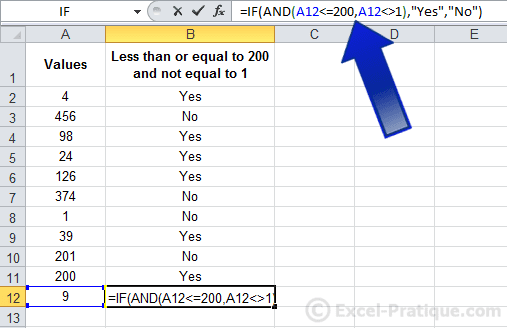# Excel Course: Comparison Operators

In the previous example, we wanted to know whether the value was "greater than", using the `>` sign. These are the other operators that can be used in its place:

 = is equal to <> is different than < is less than <= is less than or equal to > is greater than >= is greater than or equal to

As well as:

 AND =AND(formula_1,formula_2,formula_3)All the conditions must be true OR =OR(formula_1,formula_2,formula_3)At least one of the conditions must be true

Here is an example of the IF function using "AND":## Other Functions

You can find explanations of the most commonly used functions on the Functions page (which you can reach by clicking the "Functions Explained" link at the top of the page).

## Exercise

Here is an exercise in which you can practice what you have learned in this lesson: exercise6.xlsx